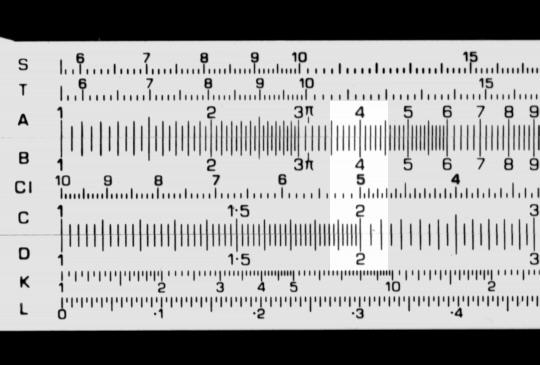Squares and square roots

This applies to rules with A and B scales.

This is normally one of the simplest functions on a slide rule. To find the square of a number:

• Move the cursor to the number on the D (or C) scale.
• Read the square of the number on the A (or B) scale.

To find the square root, work from the A (or B) scale to the D (or C) scale.

The example below shows 2 on the D scale and its square, 4, on the A scale.